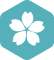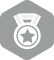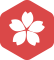### 关于数字用逗号分隔的正则表达式

Web 开发 > JavaScript [问题点数：40分，结帖人sq906224479]2013年8月 Web 开发大版内专家分月排行榜第二
2013年1月 Web 开发大版内专家分月排行榜第二JRudy

s='12345678922.12'.replace(/(\d)(?=(\d{3})+(?!\d))/g,'\$1,') console.log(s); 转载于:https://www.cnblogs.com/web-fusheng/p/8616819.html

checkNumber (number) { if (number === '') { return true } let reg = new RegExp('^(\\d{5})+(,\\d{5})*\$') let isFormatRight = reg.test(number) if (!isFormatRight) { return false ...

String[]result=orgStr.split(",");

^-?([0-9]+|[0-9]{1,3}(,[0-9]{3})*)(.[0-9]{1,2})?\$

js 正则表达式 匹配除汉字，字母，数字逗号，句号外的特殊字符（用来规范输入内容）

/[^\u4e00-\u9fa5a-zA-Z\d,\.，。]+/ 其中\u4e00-\u9fa5匹配汉字，a-zA-Z匹配字母，\d匹配数字，,.，。匹配逗号和句号，^表示非，+表示尽可能多的匹配。

var a = 222122122.6754; var b = a.toFixed(2).replace(/(\d)(?=(\d{3})+\.)/g, '\$1,');//使用正则替换，每隔三个数加一个','

‘12,34,567’ （逗号之间只有两位数字） ‘1234’ （缺少逗号） 代码如下 import re numRegex = re.compile(r'\d{1,3}(,\d{3})*\$') str = "345,456" a = numRegex.search(str) print(a.group()...

MySql 查询以逗号分隔的字符串的方法（正则

NND，一点思路都木有，想了老久，突然想到了正则表达式....！啊哈哈哈..... 1、最开始想到的是find_in_set()... 查询字段中包含01，07，08的记录： SELECT * FROM test WHERE find_in_set('01',itemn

^((([a-z0-9_\.-]+)@([\da-z\.-]+)\.([a-z\.]{2,6}\;))*(([a-z0-9_\.-]+)@([\da-z\.-]+)\.([a-z\.]{2,6})))\$

Python中利用正则表达式用逗号分割数据，但是忽略引号中的逗号的方法

public class RegexTest { public static void main(String[] args) { if("a:".matches(":\$"))...判断一个英文单词是不是以标点符号结尾，了边界匹配器\$，这里运行的结果却是没有匹配，请问这是为什么 正则

js 正则验证多个ip地址并用逗号分隔

//检测多个ip地址用逗号分隔 function checkIpv6(ip) { var reg = /^(\d{1,2}|1\d\d|2[0-4]\d|25[0-5])\.(\d{1,2}|1\d\d|2[0-4]\d|25[0-5])\.(\d{1,2}|1\d\d|2[0-4]\d|25[0-5])\.(\d{1,2}|1\d\d|2[0-4]\...

c++ 使用正则表达式分割字符串

#include #include #include int main(){  std::string s = "who,lives:in-a,pineapple under the sea?";  boost::regex re(",|:|-|\

JS基于正则实现数字千分位用逗号分割

qt正则表达式应用于文本文件分割[同时识别逗号分割符和空格等]

QStringList list = line.split(QRegExp("\\b(, | \\s+ )\\b"), QString::SkipEmptyParts);

java 正则表达式判断是否是 1到4 由逗号分隔的字符串

[code="java"] String reg = "^([1-4]+[,])*([1-4]+)\$"; Pattern pattern=Pattern.compile(reg); String str="1,2,3,3"; Matcher isNum=pattern.matcher... System.out.pri...

.NET Conf China 2020讲师 PDF

.NET Conf China 2020讲师 PDF